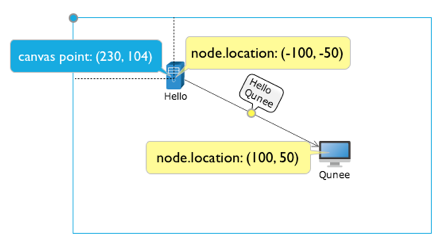Light High Efficiency Graph Component

# Graph Coordinate System

The canvas takes the central point of component as the original coordinate. This means the node of coordinate (0,0) will be presented at the centre of component. In case of no limitation on coordinate at the top left corner. Supports negative coordinate and vector zooming. In addition, the canvas range adopts the way of navigation panel (indicating the element scope by four buttons, top, bottom, left and right). Avoids the interference to the interface from rolling bar, and makes the roaming interaction more smooth.

### Coordinate origin

The initial default canvas coordinate origin at the center of the component, which means that the node (0, 0) coordinates will be presented in the central module, if you want the initial component origin is located in the upper left corner, you can set the following parameters

#originAtCenter

Set the upper left corner of the origin of coordinates

``````graph.originAtCenter = false;
``````

## Coordinate transformation

It includes canvas coordinate and logical coordinate. The previous one take the top left corner of the component interface as the original point. And the later one is the actual coordinate property of element• #globalToLocal(evt) → {Point} - calculates the relative position between the mouse point and the component according to the mouse event object.
• #toCanvas(x, y) → {Q.Point} - logical position is converted to a canvas coordinate
• #toLogical(x, y) → {Q.Point} - Canvas coordinate is converted to logical position

### Example

Monitors click event. Acquires the information about mouse point position and converts to logical coordinate

``````graph.onclick = function(evt){
var p = graph.globalToLocal(evt);
var l = graph.toLogical(p.x, p.y);
Q.log('canvas location: ' + p.x + ', ' + p.y);
Q.log('logical location: ' + l.x + ', ' + l.y);
}
``````